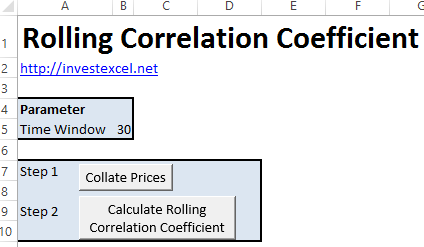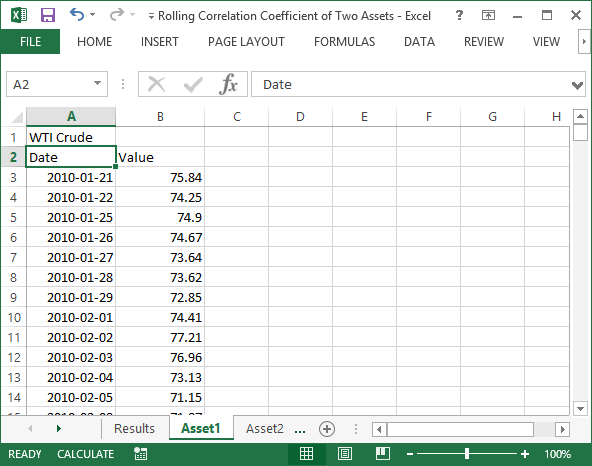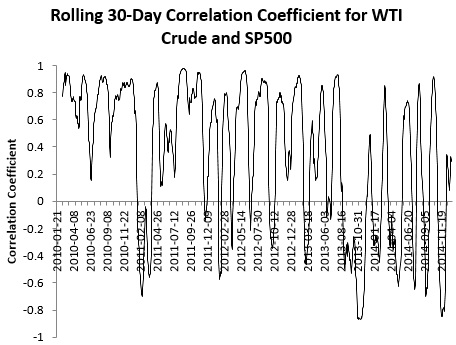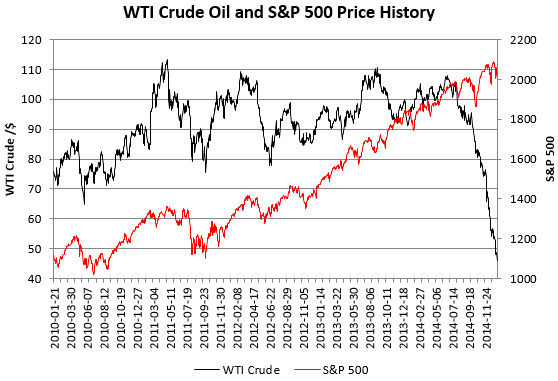# Calculating the Correlation Between the S&P 500 and Crude Oil

Learn how I calculated the rolling correlation of two key players in my retirement fund, and get a calculation spreadsheet.

In common with most investors, I want a diversified portfolio. If one of my investments drops like a brick, I don’t want everything else to crash as well.

That’s why I keep tabs on the correlation between investment pairs.

This article describes how I calculated the rolling correlation coefficient (over a customizable time period) of two major funds in my RRSP:

• a S&P 500 tracker
• and an ETF that tracks crude oil pricesIt also contains with five years of historical prices for WTI and the S&P 500. You can use the spreadsheet to analyze the relationship of your own two investments by simply copying the historical data into the appropriate tabs.

## Step 1: Getting the Historical Data

My go-to source for historical market data is Yahoo Finance. With this spreadsheet, I downloaded daily data for the S&P 500 (ticker: ^GSPC) over the last five years (January 2010 to January 2015)

However, Yahoo doesn’t give historical crude oil prices. For that, I used a spreadsheet that queries the Quandl API to download historical market prices for WTI (West Texas Intermediate) crude, again for the last five years.

The spreadsheet contains both data sets, each in a separate tab (“Asset1” and “Asset2”)If you want to copy your own data in, ensure that

• cell A1 contains the name of the stock as you want it presented in the charts,
• the time series data is copied below the data and value headers,
• and you do not change the name of the tabs (Asset1 and Asset2)

## Step 2: Aligning the Data

There are a few dates for which only the price of WTI or S&P 500 is available (but not both).

But if you want to calculate the correlation coefficient, both data sets must contain a price for every date under consideration. So I wrote some VBA that

1. calculates which data set has the shortest time history, and copies it into a new sheet – in this case it was WTI crude.
2. for each date-value pair of WTI crude, locates the price of S&P 500 with the same date.
3. place that price next to the corresponding price of oil
4. run down the partially-merged list, and delete rows for which only prices for WTI or S&P 500 is available.

There are more sophisticated methods of aligning the data, but this is quick to implement.

Here’s part of the VBA (the complete code is in the spreadsheet).

``` 'Find the asset with the shortest time history minRow = Sheets("Asset1").UsedRange.Rows.Count Set minTickerWS = Sheets("Asset1") If Sheets("Asset2").UsedRange.Rows.Count < minRow Then minRow = Sheets("Asset2").UsedRange.Rows.Count Set minTickerWS = Sheets("Asset2") End If   Sheets.Add After:=Sheets(Sheets.Count) ActiveSheet.Name = "Collated Prices"   i = 1 minTickerWS.Range("A2", "B" & minRow).Copy Destination:=Sheets("Collated Prices").Cells(1, i) Sheets("Collated Prices").Cells(1, i + 1) = minTickerWS.Range("A1")   i = i + 2   For Each ws In Worksheets If ws.Name <> "Collated Prices" And ws.Name <> minTickerWS.Name And ws.Name <> "Results" Then Sheets("Collated Prices").Cells(1, i) = ws.Range("A1") Sheets("Collated Prices").Range(Sheets("Collated Prices").Cells(2, i), Sheets("Collated Prices").Cells(minRow - 1, i)).Formula = _ "=vlookup(A2," & ws.Name & "!A\$2:G\$" & minRow & ",2,0)" i = i + 1 End If Next   nRows = Sheets("Collated Prices").Range("A" & Sheets("Collated Prices").Rows.Count).End(xlUp).Row   For i = 2 To nRows If IsEmpty(Sheets("Collated Prices").Range("B" & i)) Or IsEmpty(Sheets("Collated Prices").Range("C" & i)) Then Sheets("Collated Prices").Rows(i).EntireRow.Delete End If Next```

Click the “Collate Prices” button on the “Results” tab to run the filtering code.

## Step 3: Calculating the Correlation Coefficient

I wanted to calculate the rolling correlation coefficient over a time period of n days.

• Starting from the nth day, the code calculates the correlation coefficient for day n and the previous n-1 days
• every day, the window is moves forward one day.

There are several ways to write the formula, but I chose to use the Formula R1C1 method to programmatically place formulas into the spreadsheet. Here’s a code snippet.

```timeWindow = Sheets("Collated Prices").Range("timeWindow") Sheets("Collated Prices").Range("D" & 1 + timeWindow & ":D" & nRows - 1).FormulaR1C1 = _ "=CORREL(R[-" & timeWindow & "]C[-2]:RC[-2], R[-" & timeWindow & "]C[-1]:RC[-1])"```

Change the time window and run the complete code by clicking the “Calculate Rolling Correlation Coefficient” button in the “Results”.

## The Results!

This chart gives the rolling 30-day correlation coefficient for the S&P 500 and WTI crude between 21st January 2010 and 12th January 2015.A value of 1 means both are synchronized with each other, a value of -1 means that if one falls, the other rises, while zero means no relationship exists.

Over the last five years, the rolling correlation coefficient dips and rises fairly regularly. Increasing the time window of the rolling correlation coefficient smooths out frequency. Try experimenting with the spreadsheet and see what difference increasing or decreasing the time window makes.

The correlation coefficient over the full five years is 0.285. That’s a moderately positive correlation.

This chart gives the price of a barrel of WTI crude oil and the S&P 500 between 21st January 2010 and 12th January 2015.Both investments track upwards for the first four years (with crude oil having a rockier ride).

As you can see, something drastic happens during the latter half of 2014. WTI crude rapidly falls in price as a result of Saudi Arabia flooding the market with crude.### 4 thoughts on “Calculating the Correlation Between the S&P 500 and Crude Oil”

1. assuming your data series are in Column A and B, and start in row 2, why didn’t you just do something like the following, and drag it down:

=CORREL(OFFSET(A2,0,0,30),OFFSET(B2,0,0,30))